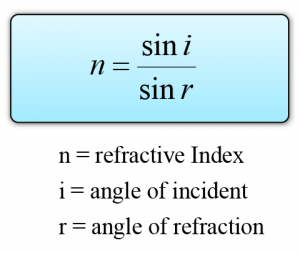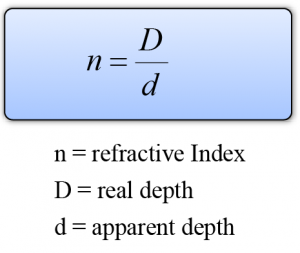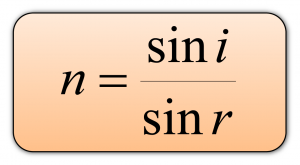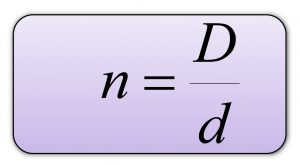# Refractive Index

### Refractive index

1. The value of  sini/sinr is called the refractive index of the medium and it gives you an indication of its light-bending ability.2. In SPM, when we say “refractive index”, what we mean is the absolute refractive index of a substance. The absolute refractive index of a substance is the refractive index where light ray travels from the vacuum (or air) into the substance.

### Refractive Index and the Speed of light( Note that the greater the refractive index of a medium, the lower is the speed of light. The more light is slowed, the more it is bent. )

### Real and Apparent Depth

1. The bending of light can give you a false impression of depth.
2. Figure to the left shows two rays of light leaving a point on the bottom of a swimming pool.
3. The rays are refracted as they leave the water. To the observer, the rays seem to come from a higher position, and the bottom looks closer to the surface than it really is.
4. The real depth of the water and its apparent depth are marked on the diagram. These are related to the refractive index of the water by the following equation:#### Summary:

Refractive index